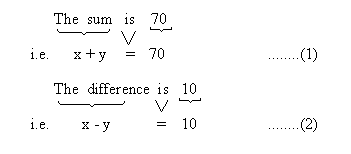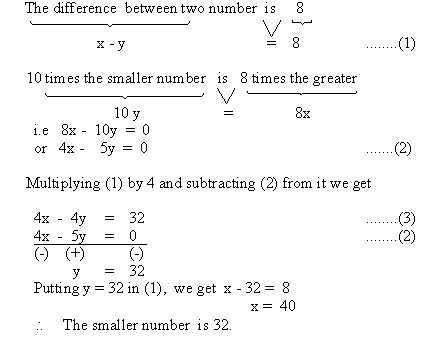Home MonkeyNotes Printable Notes Digital Library Study Guides Study Smart Parents Tips College Planning Test Prep Fun Zone Help / FAQ How to Cite New Title Request

 9.2 Use of Simultaneous Linear Equations A) Number Problems : Example Find two numbers whose sum is 70 and difference is 10. Solution : You are looking for two numbers. Let the two numbers be x and y. (x > y) Now we set up two equations from the two conditions given in the problem.Solving (1) and (2) by adding them x + y = 70 x - y = 10 ___________ 2x = 80           \ x = 40 Now, substituting in (1), we get 40 + y = 70 \ y = 30 Therefore, the two numbers are 40 and 30. Example The difference between two numbers is 8 and 10 times the smaller number and is equal to eight times the greater. Find the smaller number. Solution : Let the greater number be x and the smaller number be y. From the given conditions we set two equations asIndex 9.1 - Definition and Solving Techniques 9.2 - Use of Simultaneous Linear Equations Chapter 1
 Search: All Products Books Popular Music Classical Music Video DVD Toys & Games Electronics Software Tools & Hardware Outdoor Living Kitchen & Housewares Camera & Photo Cell Phones Keywords: